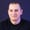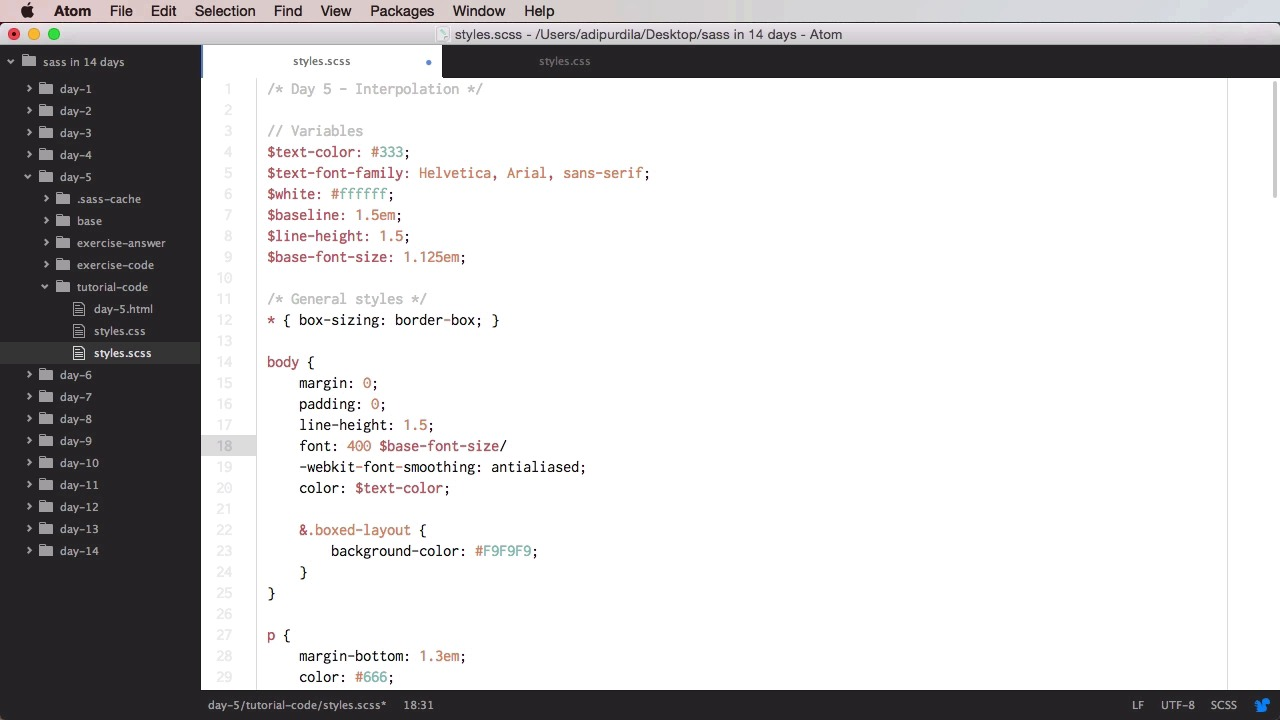Lessons: 20Length: 1.9 hours• Overview
• Transcript

# 3.5 Day 5: Interpolation

Welcome to day #5, where you’ll learn about "interpolation". This is also known as "variable interpolation" and it refers to replacing one or more variables in an expression with their value. It might seem complicated, but it’s not really. So, let’s go ahead and see some examples in Sass.

## 5.Conclusion1 lesson, 00:54

### 3.5 Day 5: Interpolation

Welcome to day five, where you'll learn about something called interpolation, or variable interpolation, and this is a technique that allows you to substitute a variable in an expression with its value. It might seem complicated, but it's not really, so let's get started. In the previous day, we've used variables as property values. But the thing is, you can also use them in selectors. And property names, but you must use a special kind of syntax, or a method called interpolation. So let's see how you would do that. First of all, let's start with a simple demo. Let's say that you have a variable called child, and this one has a value of widget. And let's say that you wanna add this variable value to a selector. For example, sidebar dash widget. Well, you cannot do sidebar dash child. This is not a valid code. Instead, what you need to do, is you need to place this between two curly brackets and a diaz sign. So, you would do diaz, curly brackets, and then inside you would do the variable name. So this will essentially replace this bit with this bit, with value of the variable. So in here, we can do stuff like, let's say, padding, and we'll use a variable that we've already defined, baseline. So notice that when you use the variable as a property value, you don't need to use interpolation. Instead you just put in the variable name. So this bit will compile to sidebar dash widgets. Very, very cool. Now where else could you use this? Well, there are lots of uses for it. For example, let's say that, let's, let's actually add a few more variables here, like. Line height, let´s say 1.5 and let´s add a base font size 1.125 m´s. And let's say that we want to use the shorthand version of the font that you saw in a previous lesson. So we would delete these and we would say something like this, font: 400. And that's the font weight, and then you would do base-font-size/$line-height. And then text-font-family. But this will not be compiled. It's gonna be compiled to this, 0.75ems, and that's because when used like this, this is a division, we have the division operator here. So what we can do is, we can select this variable, use some curly brackets, use diaz, and we'll do the same for line height. So now, instead of doing division. We're going to get the value from base font size. We'll use the slash normally, and then we'll get the value from line height. So now it will compile to this bit. So this is another example where you can use interpolation. Let's see another example. Let's have a look at this site here. We have a before pseudo element. And let's say that's inside this content here. I want to add a variable. For example, site before, right. So I would do this, site before, I would go up here, I will do$cite-before and say, for example, Author, all right. And now when I look at it, this compiles to \$cite-before. And that's because it got the variable name, literally. It did not compile it as a variable. So, again you can use interpolation here. And instead of the variable name as a simple string, this will actually grab the variable value and replace it here. So now the content is Author. It's whatever we defined. Back here. So that's how you use interpolation. Now, you can use it in some more complex Sass files, like when using Sass directives, but for now, these are the basics of it. Now, for your assignment, you have a CSS file. And your assignment is to create the SCSS file that compiles into this CSS file. But the requirements is that the the button class names, color values, font family, size and line height are all taken from variables. And also, you should use as few Sass code lines as possible. Good luck. All right, and that's it for variable interpolation. Now it's time to move on to day six, where you'll learn about the different data types available in Sass.

Back to the top